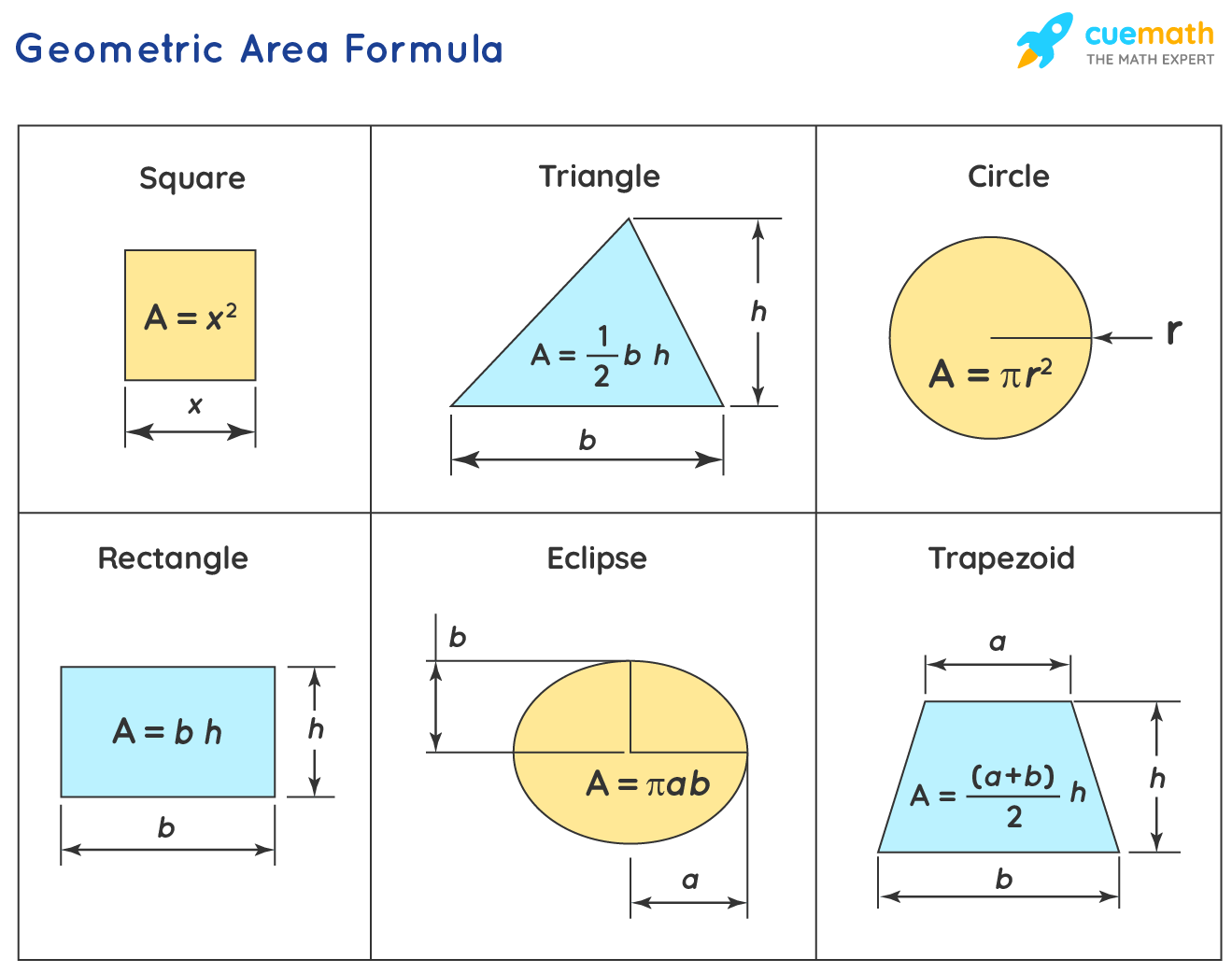# Geometric Area Formula

Go back to  'Math Formulas'

The measurement of space enclosed by a closed geometric figure is called area. Also, it is a quantity that expresses the extent of a two-dimensional figure or shape or planar lamina, in the plane. Geometric Area Formula is used to calculate the space enclosed by different geometric figures.

Let us learn geometric area formulas for different shapes using solved examples in the following sections.

## What is Geometric Area Formula?

Different Geometric Area Formulas are used to calculate the area of different geometric figures. They can be expressed as,• The formula to calculate the area of a rectangle is given as:

Area of Rectangle = (L× B) square units

where  L = length of the rectangle, B = width of the rectangle.

• The formula to calculate the area of a square is given as:

Area of Square = asquare units

where a = side of the square.

• The formula to calculate the area of a triangle is given as:

Area of a Triangle = 1/2(b×h) square units

where b = base of the triangle, h = height of the triangle.

• The formula to calculate the area of a circle is given as:

Area of a Circle = πrsquare units

where r = radius of the circle.

• The formula to calculate the area of a trapezoid is given as:

Area of a Trapezoid = 1/2 (a + b)h square units

where a, b = two parallel sides of the trapezoid, h = vertical height of the trapezoid.

• The formula to calculate the area of an ellipse is given as:

Area of Ellipse  = (πab) square units

where a = radius of the major axis, b = radius of the minor axis.

## Solved Examples Using Geometric Area Formula.

### What is the area of a rectangular park whose length and breadth are 60m and 90m respectively?

Solution:

To find: The area of a rectangular park.

Given:

Length of the park = 60m

Breadth of the park = 90m

Using Geometric Area Formula,

Area of Rectangle = (L× B)

= (60 × 90) m

= 5400 m2

Answer: The area of the rectangular park is 5400 m2.

### What is the area of a circular park whose radius is 400m?

Solution:

To find: The area of a circular park.

Given:

Radius of the circular park = 400m

Using Geometric Area Formula,

Area of a Circle = πr2

Area of a Circle = π 4002

= 160000π m2

Answer: The area of the circular park is 160000π m2.